# How to Calculate Riemann Zeta Function by Hand: A Tutorial

Knoji reviews products and up-and-coming brands we think you'll love. In certain cases, we may receive a commission from brands mentioned in our guides. Learn more.
The calculation of exact functional values of Riemann Zeta functions, zeta(n), require complicated calculations or numeric, but they can easily be approximated by doing mere calculations by hand. In this article I have discussed a very simple method of ap

The Riemann zeta function or simply zeta function is a very important mathematical function, which frequently appears in problems of physics, number theory and applied statistics. In general it is a function of a complex variable which converges when the real part of the argument (n) is greater than one. Though the exact functional values of zeta(n), which require complicated calculations or numeric, is available for a wide range of values of n, they can easily be approximated by doing mere calculations by hand. In this article I will describe a simple method of approximating zeta(n) for n>1.

The Approximated Formula:

Before going into the theory from where the formula is derived, let us first simply state it.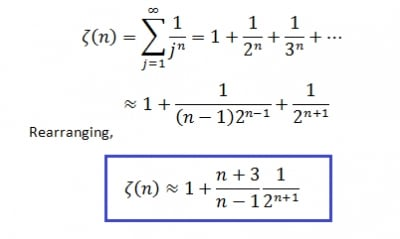As we see, the last expression for zeta(n) has only two terms and it can be easily computed by hand for integral values of n, which is often the case appearing in physics or number theory. If we need to find zeta(n) for fractional values of n, then we will have to use a scientific calculator especially to calculate the power of 2.

The Error:

Now let’s check how good this simple formula is. Here is a table providing zeta function calculated using this approximated formula, actual value of it and error in estimation for different values of n.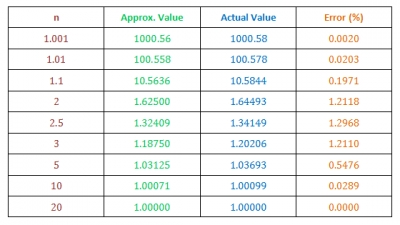Thus we see that this formula works progressively well as the value of n is increased and even for small values of n the maximum error is below 1.3%, which occurs near n=2.442. To ensure that the error falls down for all other values of n, here I give plot of percentage error vs. n. The plot asymptotically tends to zero as n goes to infinity.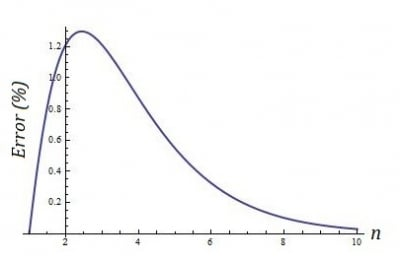Note: n=1 is not included in the table because the sum diverges in that case (as you can see there is a (n-1) term in the denominator).

The Theory:

The result follows from the Euler-Maclaurin sum formula (Ralston and Rabinowitz, 2001) which I will not discuss in details and just dictate the general formula for the infinite sum.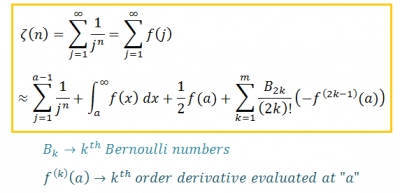Here, the sum up to n=a-1 is calculated explicitly and the last term containing Bernoulli numbers has a very small contribution to the actual sum. Normally m is taken to be 4 or 5 and the last term is considered only when higher accuracy is desired. Our formula is a special case when the parameter “a” is taken to be 2.

A “C” Code:

Here I provide a small code written in “C” utilizing the last given expression for zeta(n), excluding the last term. This indeed gives a better result but by the help of a computer; the values calculated by hand are enough accurate for regular purposes.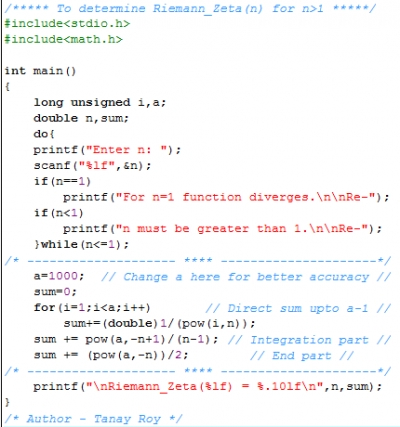Conclusion:

Thus we see that the Riemann zeta functions can be obtained with enough accuracy just by hand calculations without depending upon table of data or computer codes. This often becomes useful whenever a rough estimate of some parameter is required involving the zeta functions.